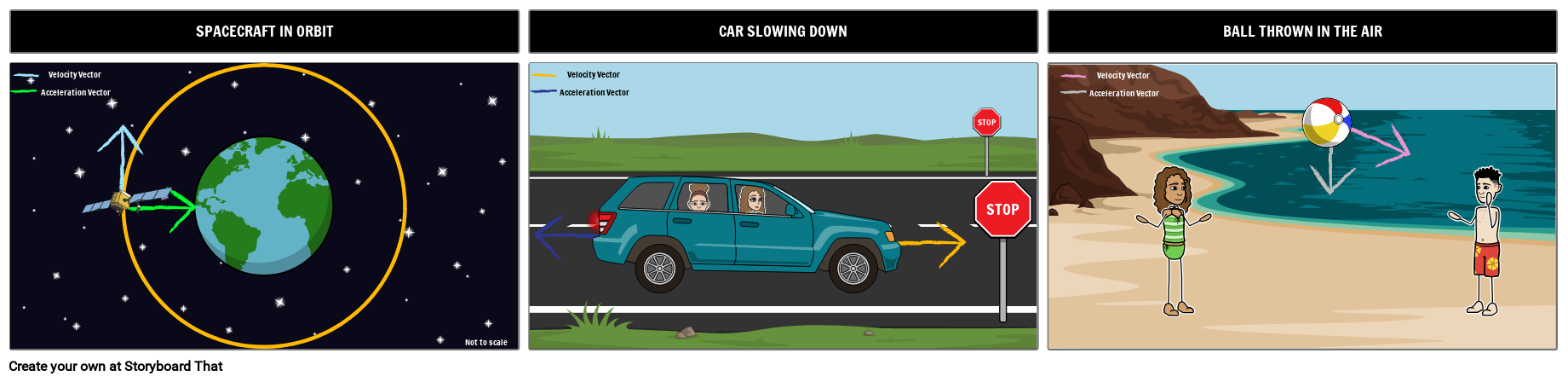## Activity Overview

Once students have mastered simple acceleration and velocity vectors (where they move in the same direction), challenge them with situations where the vectors are not in the same direction. Students often find this concept challenging, but it doesn't have to be. In this activity, students will create vector diagrams illustrating the acceleration and velocity in different situations. The suggested scenarios below are in the activity instructions, but you can choose to present students with several additional scenarios to illustrate, such as a car going around a corner or a cannon ball being fired out of a cannon.

#### Spacecraft in Orbit

The spacecraft moves in a circular path around the Earth. Its velocity vector is constantly changing, even if its speed is constant. The acceleration vector arrow points towards the center of the Earth, in the same way the force due to gravity would act.

#### Car Slowing Down

The velocity arrow changes as the car slows down. The direction of the arrow remains constant, in the direction the car is moving. The size of the velocity arrow decreases as the car gets slower. The acceleration arrow acts in the opposite direction to the velocity arrow. This is known as negative acceleration or deceleration.

#### Ball Thrown in the Air

The velocity vector points in the direction of travel and changes as the ball follows its path. The acceleration vector arrow remains constant as the ball is in the air. The arrow points directly downwards towards the Earth.

## Template and Class Instructions

(These instructions are completely customizable. After clicking "Copy Activity", update the instructions on the Edit Tab of the assignment.)

Student Instructions

Create acceleration and velocity vector diagrams for different situations.

1. Click "Start Assignment".
2. Use a combinations of scenes, characters and props to create a visualization for the following situations: spacecraft in orbit, car slowing down, and ball thrown in the air.
3. Use arrows to describe the acceleration and velocity vectors in each situation.## Rubric

(You can also create your own on Quick Rubric.)

Vector Diagrams
Draw vector diagrams for different given situations.
Proficient Emerging Beginning
Vector Arrows
All the vector arrows are in the correct direction and have the correct length.
All the vector arrows are in the correct direction.
Some the vector arrows are in the correct direction.
Vector Labels
All the vectors are correctly labeled.
Most of the vectors are correctly labeled.
A few of the vectors are correctly labeled.
Evidence of Effort
Work is well written and carefully thought out.
Work shows some evidence of effort.
Work shows little evidence of any effort.

## More Storyboard That Activities

### Motion

*(This Will Start a 2-Week Free Trial - No Credit Card Needed)